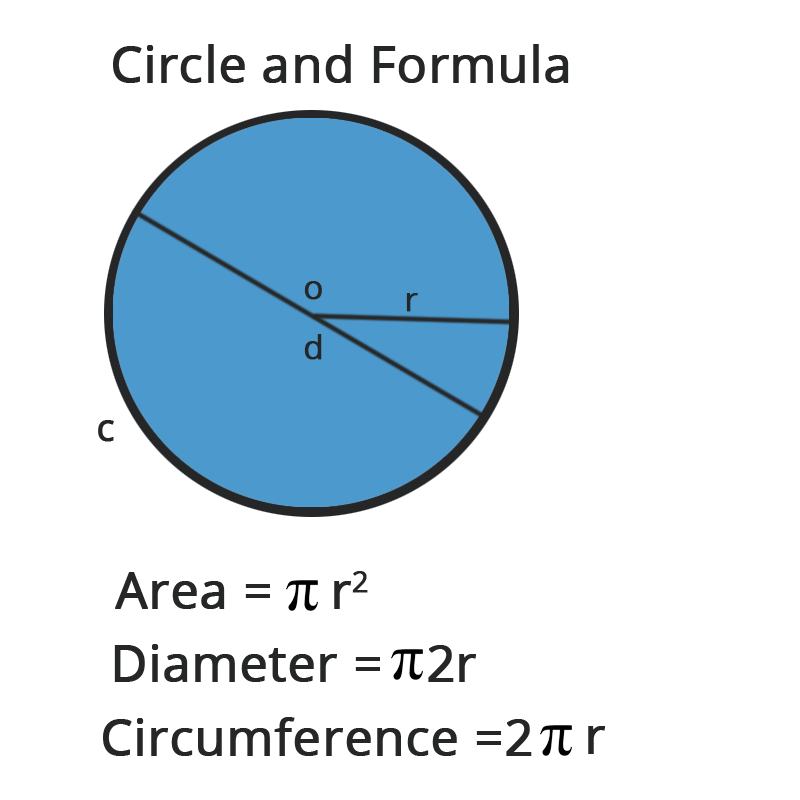• Call Now

1800-102-2727•

# Circumference of a Circle Formula, Semicircle Perimeter, Area and Examples

The circumference of any form specifies the route or border that surrounds it in mathematics. In other terms, the circumference is also known as the perimeter, and it is used to determine the length of a shape's contour. In this article, we will look at the term "circumference of a circle," including its definition, formula, and techniques for calculating the circumference of a circle, as well as numerous solved cases.

The circumference of a circle, also known as its perimeter, is the measurement of the circle's boundaries. The area of a circle, on the other hand, specifies the territory it occupies. The circumference of a circle is its length when we open it and draw a straight line through it. It's generally expressed in quantities like centimetres or meters.

The radius of the circle is taken into account when using the formula to determine the circle's circumference. As a result, to calculate the perimeter of a circle, we must first determine the radius or diameter.## The formula for the Circumference of a Circle

A circle's circumference (or perimeter) is equal to 2πR. The circle radius is R is a mathematical constant having an estimated value of 3.14 (to two decimal places). Again, Pi () is a mathematical constant representing the circumference to diameter ratio of any circle. C is equal to πD; The circumference of the circle is denoted by the letter C; The diameter of the circle is D.

### Circle's area Formula

The region contained by the circle itself, or the space covered by the circle, is the area of any circle. The formula for calculating the area of a circle is as follows:
πr2 = A
This formula applies to all circles with varying radii, where r is the radius of the circle.

### Semicircle Perimeter

When we divide a circle into two halves, we get a semicircle. As a result, the semi-perimeter circles are cut in half.

As a result, Perimeter = πr + 2r

### Semi-Circular Area

The area of a semicircle on a 2D plane is the area occupied by a semicircle. Half of the area of a circle with equal radii is equal to the area of a semicircle. The area is πr2/2.

The radius of a circle is the distance between its centre and its outside line. It is the most significant quantity in the circle, from which the formulae for the circle's area and circumference are generated. A circle's diameter is equal to twice its radius. A semicircle is created when the diameter divides a circle into two halves.

The circumference of a circle or other curved figure is defined as the distance between two points. It is the linear measurement of the outer surface of any two-dimensional circular figure.

The circumference calculation is sometimes known as the perimeter of the circle since it follows the same idea as determining the perimeter of any polygon. A circle is a form in which all of the points are equidistant from a central point.

Pi has a value of roughly 3.1415926535897... This number is represented by a Greek letter (pronounced Pi). A non-terminating value has no end.

What is a circle's circumference?
The linear distance around a circle is known as its circumference. To put it another way, if a circle is opened to produce a straight line, the length of that line equals the circle's diameter.

What is the formula for calculating the circumference of a circle?
The calculation of the circumference of a circle is simple, and you just have to multiply its diameter with the constant pi (3.142). To get the circumference from radius, multiply 2 x radius by pi.

What is the formula for calculating diameter from circumference?
Circumference = diameter * π is the formula.

Alternatively, diameter = circumference/π. As a result, the circle's diameter in terms of the circumference equals the circumference of the circle divided by pi.Talk to our expert
Resend OTP Timer =
By submitting up, I agree to receive all the Whatsapp communication on my registered number and Aakash terms and conditions and privacy policy